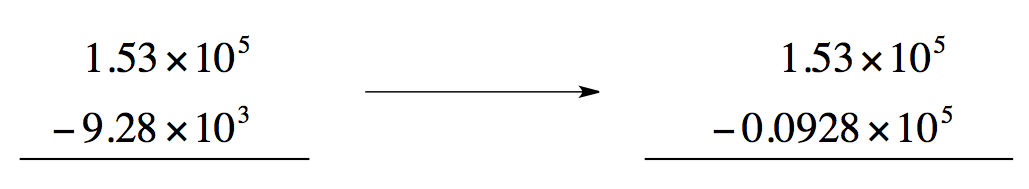Clutch Prep is now a part of Pearson
BONUS: Mathematical Operations and FunctionsWorksheetSee all chapters

# Addition and Subtraction Operations

See all sections
Sections
Multiplication and Division Operations
Addition and Subtraction Operations
Power and Root Functions
Power and Root Functions

Addition and subtraction of values in scientific notation.

###### Addition and Subtraction

Whenever we add or subtract values in scientific notation we must make sure the exponents are the same value.

Concept #1: Addition and Subtraction Operations

If the exponents are not the same then we transform the smaller value so that they do.Concept #2: Addition and Subtraction Calculations 1

Concept #3: Addition and Subtraction Calculations 2